# Modern Robotics Errata

(Difference between revisions)

Errata below are for the hardcover book published by Cambridge University Press and the preprint version of the book posted on May 3, 2017. Thanks to everyone who provided corrections to earlier versions!

## Significant corrections

### Chapter 3

• In the caption of Figure 3.3, it should read$\hat{\text{x}}_{\text{b}} = (1/2, \sqrt{3}/2)$ and$\hat{\text{y}}_{\text{b}} = (-\sqrt{3}/2, 1/2)$.
• In the displayed equation just after Equation (3.76), the left-hand side should be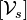$[\mathcal{V}_s]$ (the brackets are missing).

### Chapter 4

• Example 4.1, last row of the table (screw axis for joint 3): The linear component vi should be (0, − L2,0) (the sign is missing).

### Chapter 5

• Equation (5.7): The the first two terms on the right-hand side of the equation should be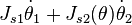$J_{s1} \dot{\theta}_1 + J_{s2}(\theta) \dot{\theta}_2$.
• Exercise 5.16 and Figure 5.26: The robot is referred to as PRRRRR, but it is PRPRRR.

### Chapter 6

• Chapter 6.3, first line after Eq (6.7): the matrices$T_{sd}^{-1} \dot{T}_{sd}$ and$\dot{T}_{sd} T_{sd}^{-1}$ are referred to as twists, but these are the se(3) matrix representations of the twists.

### Chapter 8

• Figure 8.5 says the volume of the rectangular parallelepiped is abc but it should be hlw.
• End of first sentence after Eq (8.26) in Section 8.2.1 should read "columns of Rbc correspond to the eigenvectors of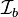$\mathcal{I}_b$." (The word "eigenvalues" should be replaced by "eigenvectors.")
• Caption of Figure 8.10: The operating region is light gray and the continuous operating region is dark gray.
• Exercise 8.6(a): The expression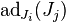$\text{ad}_{J_i}(J_j)$ has the indices switched; the correct expression is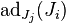$\text{ad}_{J_j}(J_i)$.
• Exercise 8.7: The expression should be written: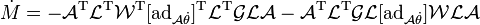$\dot{M} = -\mathcal{A}^{\rm T} \mathcal{L}^{\rm T} \mathcal{W}^{\rm T} [\mbox{ad}_{\mathcal{A} \dot{\theta}}]^{\rm T} \mathcal{L}^{\rm T} \mathcal{GLA} - \mathcal{A}^{\rm T} \mathcal{L}^{\rm T} \mathcal{GL} [\mbox{ad}_{\mathcal{A} \dot{\theta}}] \mathcal{WLA}$

### Chapter 10

• Equation (10.4) should read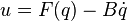$u = F(q) - B \dot{q}$ (the plus sign in the book should be a minus sign).

### Chapter 11

• In the displayed equation after Equation (11.18), the vector Xe(t) is a six-vector. The bottom three elements are written correctly, but the top three elements, an angular velocity, are written instead in their 3x3 so(3) form.
• In Equation (11.33), the right-hand side should be zero (c = 0).
• Figure 11.24, the Robonaut 2 series elastic actuator, 4th sentence of the caption: The words "outer" and "inner" should be switched, so the new sentence reads "The inner ring of hole mounts connects to the harmonic gearhead output, and the outer ring of hole mounts is the output of the SEA, connecting to the next link."

### Appendix C

• Section C.3, Equation (C.5): All instances of ϕi − 1 should be replaced by ϕi.

## Minor typos, etc., no danger of misunderstanding

### Chapter 1

• (online version only) Description of Chapter 6: "jont positions" should be "joint positions."

### Chapter 2

• Chapter 2.2.2, Example 2.3: "Substituting" is misspelled.

### Chapter 6

• (online version only) Figure 6.7: θ0 should be θ0 (five times) and θ1 should be θ1 (one time).

### Chapter 7

• Just below caption for Figure 7.8: There is an extraneous dot.

### Chapter 8

• (online version only) Chapter 8.1.1, last paragraph: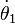$\dot{\theta}_1$ is improperly typeset.

### Chapter 12

• Caption of Figure 12.14, second-to-last sentence: "rotation if possible" should be "rotation is possible."

## A partial list of errata contributors

Thanks to the following people who provided corrections, starting from the preliminary version of the book posted in October, 2016:

H. Andy Nam, Eric Lee, Yuchen Rao, Chainatee Tanakulrongson, Mengjiao Hong, Kevin Cheng, Jens Lundell, Elton Cheng, Michael Young, Jarvis Schultz, Logan Springgate, Sofya Akhmametyeva, Aykut Onol, Josh Holcomb, Yue Chen, Mark Shi, AJ Ibraheem, Yalun Wen, Seongjae Jeong, Josh Mehling, Felix Wang, Drew Warren, Chris Miller, Clemens Eppner, Zack Woodruff, Jian Shi, Jixiang Zhang